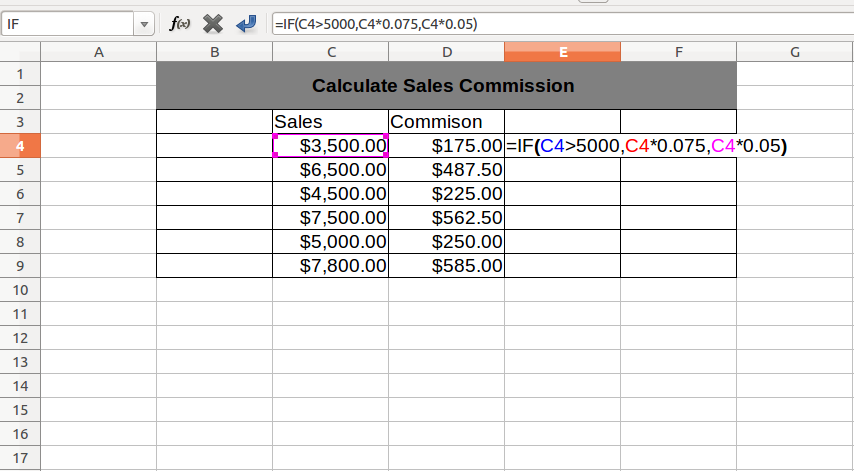IF Function in Excel : In this excel tutorial we will learn about syntax, usage and nesting of IF function.

## Description:

The IF function is the most popular function for a logical test in Excel worksheet. It compares two values and performs a specific task based on given TRUE or FALSE value.

## Syntax:

=IF(logical_test, [value_if_true], [value_if_false])

• logical_test – Boolean value, condition or logical expression which returns TRUE or FALSE.
• value_if_true – The task which you want to perform if the condition is TRUE [optional].
• value_if_false – The task which you want to perform if the condition is FALSE [optional].

## Note:

[optional] – optional means not a compulsory argument, if you need you can put task or can leave blank.

## Example:

### Example 1: Find largest number.

In the above example, we compare two numbers and print largest one. If the condition is TRUE then it will print First and if the condition is FALSE then it will print Second.

In the above example IF function will print the largest number (Cell Value), based upon given condition.

### Example 2: Calculate sales commission based on revenue.Above example show common calculation to show sales commission based on revenue generated by sales person.

## Conclusion :

So far we have learned about Syntax and how to use IF function in Excel. In IF formula as a value (Either True Or False) we can use :

• Any Text value
• Cell reference
• Calculation or Expression
• Formula also.

IF Function in Excel